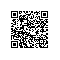# More Effective C++ 读书笔记二

#include <iostream>
class A
{
public:
A(int a){_a = a;}
bool operator==(const A &lhs) {return _a == lhs._a;}
private:
int _a;
};int main()
{
A a(1);
if(a == 1)
std::cout << “equal”;
else
std::cout << “not equal”;
return 0;
} [/cce]

test.cpp: 在函数‘int main()’中:
test.cpp:15:10: 错误：‘operator==’在‘a == 1’中没有匹配
test.cpp:7:7: 附注：备选是： bool A::operator==(const A&)使用钉钉扫一扫加入圈子
+ 订阅